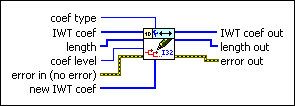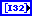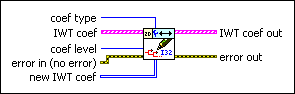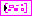# WA Set Coefficients of Integer Wavelet Transform VI

LabVIEW 2014 Advanced Signal Processing Toolkit Help

Edition Date: June 2014

Part Number: 372656C-01

»View Product InfoDownload Help (Windows Only)

Owning Palette: Discrete Wavelet VIs

Sets approximation or detail integer wavelet transform (IWT) coefficients at a specific level. Use the WA Integer Wavelet Transform VI to compute the IWT. Use the WA Get Coefficients of Integer Wavelet Transform VI to obtain the IWT coefficients.

Use the pull-down menu to select an instance of this VI.

 Select an instance WA Set Coefficients of 1D Integer Wavelet TransformWA Set Coefficients of 2D Integer Wavelet Transform

## WA Set Coefficients of 1D Integer Wavelet Transformcoef type specifies whether this VI sets the approximation coefficients or detail coefficients. The default is Approximation coefficients.IWT coef specifies the approximation coefficients and the detail coefficients from the multi-level integer wavelet transform (IWT). You must organize the coefficients into a 1D integer array starting with the approximation coefficients at the largest level followed by the detail coefficients at all levels in descending order. If you use the WA Get Coefficients of Integer Wavelet Transform VI to obtain the IWT coefficients, wire the IWT coef out output of the WA Get Coefficients of Integer Wavelet Transform VI to the IWT coef input of this VI.length specifies, in a 1D array, the number of approximation and detail coefficients at each level. At a decomposition level of N, length is equal to N+2. The first element of length always is equal to the number of approximation coefficients. The last element of length indicates the total number of raw data samples. You must arrange the length of the detail coefficients in descending order. If you use the WA Get Coefficients of Integer Wavelet Transform VI to obtain the IWT coefficients, wire the length out output of the WA Get Coefficients of Integer Wavelet Transform VI to the length input of this VI.coef level specifies the coefficient level this VI sets. The coef level must be between 1 and the largest level of the detail coefficients. The levels in the WA Integer Wavelet Transform VI specifies the largest level of the coefficients. The default is 1, which means this VI sets the coefficients at level 1. If coef type is Approximation coefficients, this VI ignores coef level. If coef type is Detail coefficients, this VI sets the coefficients at the level you specify in coef level.error in describes error conditions that occur before this node runs. This input provides standard error in functionality.new IWT coef specifies the integer wavelet transform (IWT) coefficients to set.IWT coef out returns the integer wavelet transform (IWT) coefficients set to new IWT coef at the coef type and coef level.length out returns length unchanged.error out contains error information. This output provides standard error out functionality.

## WA Set Coefficients of 2D Integer Wavelet Transformcoef type specifies the coefficient type this VI sets.

 0 low_low (default)—Specifies the approximation coefficients from the lowpass analysis filtering on each row and each column. The low_low coefficients are a low-resolution approximation of the original 2D signal. 1 low_high—Specifies the detail coefficients from the lowpass analysis filtering on each row and the highpass analysis filtering on each column. The high-frequency signal along the column direction influences the low_high coefficients. 2 high_low—Specifies the detail coefficients from the highpass analysis filtering on each row and the lowpass analysis filtering on each column. The high-frequency signal along the row direction influences the high_low coefficients. 3 high_high—Specifies the detail coefficients from the highpass analysis filtering on each row and each column. The high-frequency signal along the diagonal direction influences the high_high coefficients.IWT coef specifies the approximation coefficients and the detail coefficients from the multi-level integer wavelet transform (IWT). Each element of the array contains the 2D IWT results of one level. The ith element stores the approximation coefficients and the detail coefficients at level i+1. If you use the WA Get Coefficients of Integer Wavelet Transform VI to obtain the IWT coefficients, wire the IWT coef out output of the WA Get Coefficients of Integer Wavelet Transform VI to the IWT coef input of this VI.low_low specifies the approximation coefficients from the lowpass analysis filtering on each row and each column. The low_low coefficients are a low-resolution approximation of the original 2D signal.low_high specifies the detail coefficients from the lowpass analysis filtering on each row and the highpass analysis filtering on each column. The high-frequency signal along the column direction influences the low_high coefficients.high_low specifies the detail coefficients from the highpass analysis filtering on each row and the lowpass analysis filtering on each column. The high-frequency signal along the row direction influences the high_low coefficients.high_high specifies the detail coefficients from the highpass analysis filtering on each row and each column. The high-frequency signal along the diagonal direction influences the high_high coefficients.coef level specifies the coefficient level this VI sets. The coef level must be between 1 and the largest level of the coefficients. The levels in the WA Integer Wavelet Transform VI specifies the largest level of the coefficients. The default is 1, which means this VI sets the coefficients at level 1.error in describes error conditions that occur before this node runs. This input provides standard error in functionality.new IWT coef specifies the integer wavelet transform (IWT) coefficients to set.IWT coef out returns the integer wavelet transform (IWT) coefficients set to new IWT coef at the coef type and coef level.low_low out returns the approximation coefficients from the lowpass analysis filtering on each row and each column. The low_low out coefficients are a low-resolution approximation of the original 2D signal.low_high out returns the detail coefficients from the lowpass analysis filtering on each row and the highpass analysis filtering on each column. The high-frequency signal along the column direction influences the low_high out coefficients.high_low out returns the detail coefficients from the highpass analysis filtering on each row and the lowpass analysis filtering on each column. The high-frequency signal along the row direction influences the high_low out coefficients.high_high out returns the detail coefficients from the highpass analysis filtering on each row and each column. The high-frequency signal along the diagonal direction influences the high_high out coefficients.error out contains error information. This output provides standard error out functionality.